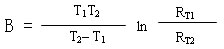﻿ What is the B value of the NTC thermistor?_

China sensor manufacturers

# What is the B value of the NTC thermistor?

B value is the constant value of NTC thermistor. That is, NTC thermistor chip (semiconductor ceramic) after high temperature sintering, Forming a negative temperature coefficient material having a resistivity. Different formulations and sintering temperature of the formation of the resistance, have different B value,So called the material constant. Or called thermal index.
NTC thermistor that B value is how to calculate?
Temperature coefficient refers to the temperature rise of 1 degree ,Resistance rate of change. Convert the value of B into the resistance temperature coefficient formula:
Thermistor temperature coefficient = B value / T ^ 2 (T is the absolute temperature of the point to be converted)
At the same temperature,The larger the B value,The smaller the resistance.
1>, B value calculation formula:
T1 / T2 is generally 25/85 or 25/50 or 25/100, depending on the definition of different manufacturers
R1 = the resistance value at temperature T1
R2 = resistance at temperature T2
T1 = 298.15K (273.15 + 25 ℃) is defined as Kay temperature
T2 = 358.15K (273.15 + 85 ℃) is defined as Kay temperature
Resistance with temperature changes in the heat sensitive index, The unit is K. This parameter is similar to the slope of the NTC product RT curve, The greater the value, Indicating that every 1 ℃ rise in temperature, The greater the change in resistance.﻿
﻿